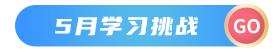# 1. csv文本概述

CSV文件是一种纯文本文件，以`逗号`分隔数值的文件类型，可以使用任何文本编辑器进行编辑，它支持追加模式，节省内存开销。在数据库或电子表格中，常见的导入导出文件格式就是CSV格式。

# 2. 操作CSV文件

csv文件内容：`````` import pandas as pd
path= 'D:\\test.csv'
with open(path)as file:
print(data)
``````
``````结果演示：
姓名  年龄   职业  家庭地址     工资
0     张三  22   厨师   北京市   6000
1     李四  26  摄影师  湖南长沙   8000
2     王五  28  程序员    深圳  10000
3   Kaina  22   学生 黑龙江   2000
4     曹操  28   销售    上海   6000
``````

## 2.1 describe()方法数据统计

``````import pandas as pd
path= 'D:\\test.csv'
with open(path)as file:
print(data.describe())
``````
``````结果演示：
年龄            工资
count   5.00000      5.000000
mean   25.20000   6400.000000
std     3.03315   2966.479395
min    22.00000   2000.000000
25%    22.00000   6000.000000
50%    26.00000   6000.000000
75%    28.00000   8000.000000
max    28.00000  10000.000000
``````

## 2.2 读取文件前几行数据

``````import pandas as pd
path= 'D:\\test.csv'
with open(path)as file:
#读取前2行数据
``````
``````结果演示：
姓名  年龄   职业  家庭地址    工资
0  张三  22   厨师   北京市  6000
1  李四  26  摄影师  湖南长沙  8000
``````

## 2.3 读取某一行所有数据

``````import pandas as pd
path= 'D:\\test.csv'
with open(path)as file:
#读取第一行所有数据
print(data.ix[0,])
``````
``````结果演示：

``````

## 2.4 读取某几行的数据

``````import pandas as pd
path= 'D:\\test.csv'
with open(path)as file:
#读取第一行、第二行、第四行的所有数据
print(data.ix[[0,1,3],:])
``````
``````结果演示：
姓名  年龄   职业  家庭地址    工资
0     张三  22   厨师   北京市  6000
1     李四  26  摄影师  湖南长沙  8000
3  Kaina  22   学生   黑龙江  2000
``````

## 2.5 读取所有行和列数据

``````import pandas as pd
path= 'D:\\test.csv'
with open(path)as file:
#读取所有行和列数据
print(data.ix[:,:])
``````
``````结果演示：
姓名  年龄   职业  家庭地址     工资
0     张三  22   厨师   北京市   6000
1     李四  26  摄影师  湖南长沙   8000
2     王五  28  程序员    深圳  10000
3  Kaina  22   学生   黑龙江   2000
4     曹操  28   销售    上海   6000
``````

## 2.6 读取某一列的所有行数据

``````import pandas as pd
path= 'D:\\test.csv'
with open(path)as file:
# print(data.ix[:, 4])
print(data.ix[:,'工资'])
``````
``````结果演示：
0     6000
1     8000
2    10000
3     2000
4     6000
Name: 工资, dtype: int64
``````

## 2.7 读取某几列的某几行

``````import pandas as pd
path= 'D:\\test.csv'
with open(path)as file:
print(data.ix[[0,1,3],['姓名','职业','工资']])
``````
``````结果演示：
姓名   职业    工资
0     张三   厨师  6000
1     李四  摄影师  8000
3  Kaina   学生  2000
``````

## 2.8 读取某一行和某一列对应的数据

``````import pandas as pd
path= 'D:\\test.csv'
with open(path)as file:
#读取第三行的第三列
print("职业---"+data.ix[2,2])
``````
``````结果演示：职业---程序员
``````

# 3. CSV数据的导入导出(复制CSV文件)

``````import pandas as pd
#1.读入数据
``````

``````import pandas as pd
#1.写出数据，目标文件是Aim.csv
data.to_csv('Aim.csv')
``````

``````import pandas as pd
#填写url读取
``````

# 4. 实例演示

`test.csv`原文件内容``````import pandas as pd
file=open('test.csv')
#1.读取file中的数据
#2.把data写到目标文件Aim.csv中
data.to_csv('Aim.csv')
print(data)
``````csv参考文档